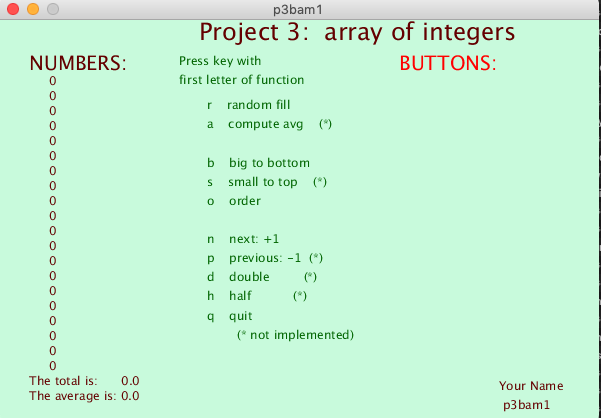## PROJECT 3: Array of integers

• Create an array of integers.

• Display the values of each element.

• Write a (void) function to display the contents of the array, one element per line.

• Compute and display the sum total and the mean average of all elements in the array. (For extra credit, comput the variance and standard-deviation.)

• Write functions to modify or manipulate ANY array of integers, performing operations as described in the table below.

• Add buttons as indicated, and add code to mousePressed() to call each function when the corresponding button is clicked.Click to enlarge.

Each function should accept two arguments:

• an array of integers
• the size of the array.
NOTE:   Do not use global variables in these functions; instead, use the arguments, as in the following example:

Here is an example of code that displays an array:
 ```int sum( int[] a, int m ) { // Display a list of array values int x=50, y=60, spacing=15; textSize( 12 ); for (int j=0; j

This function returns the sum of the elements:
 ```float sum( int[] a, int m ) { // Add 'em up! // Compute the sum; return the total. // float result=0; for (int j=0; j

These functions are called

• when the specified key is pressed, OR . . .
• when the corresponding button is clicked.
Action Key Button
reset( int a[], int m )
// Replace all numbers with random values.
r RESET
calc( int a[], int m )
// Calculate new total and average.
c CALC
next( int a[], int m )
// Increase each of the numbers by one.
n NEXT
dbl( int a[], int m )
// Double each of the numbers.
d DOUBLE
half( int a[], int m )
// Reduce each by half.
h HALF
big( int a[], int m )
// Move biggest number to end of the array.
// (Swap elements, to preserve all numbers.)
b BIG
sort( int a[], int m )
// Sort the entire array.
s SORT

NOTE:   Do not use global values in these functions; use the arguments.

KEYS   The keyPressed() function should have code to call a function when the key is the first letter of that function.
 ``` r reset() // Replace with random values. c calc() // Calculate new sum and average. n next() // Increase each by one. d dbl() // Double each of the numbers. h half() // Reduce each by half. b big() // Move biggest to end of array. s sort() // Sort the entire array. ```

BUTTONS
create buttons with names for each of these,
and add mousePressed() code to call these functions when button is pressed.